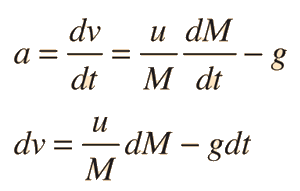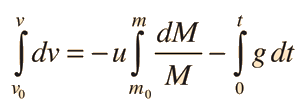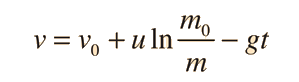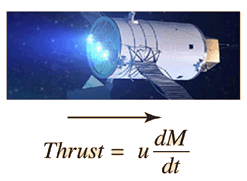# Vertical Rocket### Calculation

Under the influence of the rocket thrust and gravity, the acceleration isIntegrating from the initiation of the thrust to an arbitrary time t:gives the velocity at time t:Index

Rocket propulsion

 HyperPhysics***** Mechanics ***** Newton's laws R Nave
Go Back

# Rocket in Space

Under the influence of the rocket thrust in a gravity-free environment , the acceleration is### CalculationIntegrating from the initiation of thrust to an arbitrary time tgives the velocity at time t:The above is the standard "rocket in space" scenario where you typically calculate the velocity after a given time of thrusting in terms of the amount of fuel burned and exhausted. If you want a specific expression for velocity in terms of time, that can be developed in terms of the exhaust rate as a function of time. If the burn rate is constant at Δm/Δt then the velocity as an explicit function of time would beFor a variable burn rate, the denominator would become an integral.

Index

Rocket propulsion

 HyperPhysics***** Mechanics R Nave
Go Back

# Rocket in Space

Under the influence of the rocket thrust in a gravity-free environment, the resulting velocity can be calculated from:
First rocket equationSecond rocket equation### Develop expression

Velocity=Initial velocity + Exhaust velocity x ln(original mass /mass)
 m0 = kg
 v0 = m/s
 m = kg
 v = m/s
 The exhaust velocity
 u = m/s
To explore the effects of the various parameters on the motion of the rocket, you may substitute numbers in any of the boxes. When you have finished entering data, click on the active text above for the quantity you wish to calculate. The quantities will not be forced to be consistent until you click on the desired quantity. Note that the mass m0 includes the fuel mass, and the mass m can be considered to be the payload mass after a specified burn.
Fowles and Cassiday give as example data for a satellite launch a low orbit velocity of about 8 km/s, an initial velocity of about 0.5 km/s from the rotation of the earth near the equator, and an exhaust velocity of 2.5 km/s. You could use this data above to confirm that you could get only about 5 percent of the total initial mass into orbit. They suggest that effective ejection speeds for most rocket fuels are on the order of 2 to 4 km/s. How much would a fuel on the upper end of that ejection speed range increase your payload?
Index

Rocket propulsion

 HyperPhysics***** Mechanics R Nave
Go Back